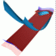Abstract and Contents
Up: 5. Examples
Next: 5.5 Normal form of the Hopf-Hopf bifurcation
Previous: 5.3 A saddle surface

# 5.4 The Lorenz system

If the Foliation Condition is not satisfied, it is still possible to compute part of the global manifold. This is illustrated with the computation of the stable manifold of the origin for the Lorenz systemWe use the standard choice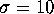,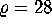andfor the parameters. The stable manifold of the origin is known to have a complicated structure, because it wraps in between the well-known Lorenz attractor, which is located around two other equilibria. As a consequence,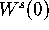spirals around the z-axis. It is best to use an algorithm designed for vector fields to compute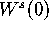, like it was done to obtain Figure 15, produced by [Worfolk 1997].

Nevertheless, we demonstrate with the computation of this manifold how our algorithm performs when the Foliation Condition is not satisfied. To this end we consider the time t map of (7) for fixed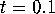, where a fourth-order Runge-Kutta method is used for the integration; see [Press et al. 1992]. We take the linear foliation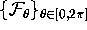, where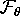is the plane perpendicular to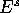, through the ray inwith argument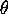. Note thatis such that any linear foliation violates the Foliation Condition. The result of the computation is shown in Figure 16.

The intersections ofwith leaves of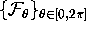are computed with an accuracy of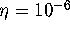. The first ring is centered at the origin and has inner radius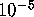and outer radius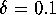. On each boundary of the ring we take 40 uniformly spaced mesh points. The width of the rings on the manifold does not exceed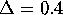, and the colored rings in Figure 16 have width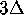. If the distance between neighboring points on different leaves exceeds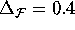, an additional leaf is added. We repeated the computation with smaller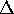and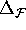, but the result was similar. The manifold in Figure 16 does not seem to intersect the attractor, which is another indication that our computation is correct.

The clockwise and counter clockwise wrapping of the manifold in the right and left half of the attractor, respectively, causeto become tangent to a foliation leaf. This is also demonstrated in the animations. The algorithm adds rings to the approximation ofuntil a piece of the manifold is missed that is necessary for the further computation. The (arclength) distance of the outer circle, that consists of 1267 points, to the origin is approximately 42.6, so that a substantial part of the stable manifold was computed.

We remark that it is best to have a mesh with triangles that intersect the leaf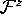through the z-axis, because the z-axis is invariant for the time t-map. The reason is computational: the image of a triangle never intersects, unless the triangle itself intersects.

Up: 5. Examples
Next: 5.5 Normal form of the Hopf-Hopf bifurcation
Previous: 5.3 A saddle surfaceAbstract and Contents

Written by: Bernd Krauskopf & Hinke Osinga
Created: May 27 1997 --- Last modified: Wed Jul 2 10:51:02 1997